# A Quick How-to on Labelling Bar Graphs in ggplot2

Monday • July 5, 2021

## Introduction

Bar charts are likely the most common chart type out there and come in several varieties. Most notably, direct labels can increase accessibility of a bar graph and reduce the “chart junk” since grid lines, axis labels, and even axis titles become obsolete. Ordering your bar charts make sense in case the categorical value has no internal order and helps focusing on the largest and smallest groups. In addition, one can highlight specific bars with use of custom colors. It is pretty easy to improve your ggplot with a few lines of code. And this short tutorial shows you multiple ways how to do so.

A few days ago, I got a request on some code creating bar charts with individual colors and percentage labels with the `{ggplot2}` package.

Watching your webinar about ggplot2 on UseR Oslo YouTube Channel, I noticed some charts you made for a project called ‘Survey on contract termination during the COVID-19 pandemic for kuendigung.org’.

Because they have many interesting aesthetic features, I started looking for source code on your GitHub repo, but I didn’t find anything. So, I kindly ask you if you can share the code you used to create those charts (or share its location), hoping that it is not copyrighted material.Specifically, the main things of interest where:

1. How to calculate the percentage values?
(Did you use a `{ggplot2}` function or calculate them manually?)
2. How to position the percentage labels inside the bars?
3. How to color the bars using different colors?

Unfortunately, the code is under lock but it’s a simple bar chart with some labels, so I will walk you through a short toy example using the manufacturers data set `mpg` that comes with `{ggplot2}`.

## Data Preparation with the tidyverse

First, let’s prepare the data for the bar chart. We are going to use the data from 2008 only and summarize the number of car model variants in the data per manufacturer. We also adjust the manufacturer labels and order them as they should appear in the final plot. Here are some notes on some of the functions used:

• `count()` from the `{dplyr}` package is a wrapper of `group_by(var) |> summarize(n = n()))`. It allows you to sort the values which is useful here because we want to order the bars based on their value in our visualization.
• `str_to_title()` from the `{stringr}` package is a quick way to capitalize labels.
• `fct_lump()`, `fct_inorder()`, `fct_rev()`, and `fct_relevel()` are all from the `{forcats}` package that provides helpers for reordering factor levels.
• First, we group all manufacturers together that do not belong to the top 10 with `fct_lump()`.
• Since our data set is sorted in descending order thanks to `count()`, we first order them by appearance with `fct_inorder()`.
• Afterwards, we reverse them with `fct_rev()`(so that the bar with the highest value is on top).
• Finally, we move the category “Other” to the end (as the first level) with `fct_relevel()`.
``````library(tidyverse)

mpg_sum <- mpg |>
## just use 2008 data
dplyr::filter(year == 2008) |>
## turn into lumped factors with capitalized names
dplyr::mutate(
manufacturer = stringr::str_to_title(manufacturer),
manufacturer = forcats::fct_lump(manufacturer, n = 10)
) |>
dplyr::count(manufacturer, sort = TRUE) |>
## order factor levels by number, put "Other" to end
dplyr::mutate(
manufacturer = forcats::fct_rev(forcats::fct_inorder(manufacturer)),
manufacturer = forcats::fct_relevel(manufacturer, "Other", after = 0)
)

mpg_sum``````
``````## # A tibble: 11 × 2
##    manufacturer     n
##    <fct>        <int>
##  1 Dodge           21
##  2 Toyota          14
##  3 Chevrolet       12
##  4 Volkswagen      11
##  5 Other           11
##  6 Ford            10
##  7 Audi             9
##  8 Hyundai          8
##  9 Subaru           8
## 10 Nissan           7
## 11 Jeep             6``````

Let’s check if our factor reordering worked:

``levels(mpg_sum\$manufacturer)``
``##   "Other"      "Jeep"       "Nissan"     "Subaru"     "Hyundai"    "Audi"       "Ford"       "Volkswagen" "Chevrolet"  "Toyota"     "Dodge"``

Keep in mind that we have reversed the ordering since `{ggplot2}` plots factors from bottom to top when being mapped to `y`.

## Data Visualization with ggplot2

First, let’s draw the basic bar chart using our aggregated and ordered data set called `mpg_sum`:

``````ggplot(mpg_sum, aes(x = n, y = manufacturer)) +
## draw bars
geom_col(fill = "gray70") +
## change plot appearance
theme_minimal()``````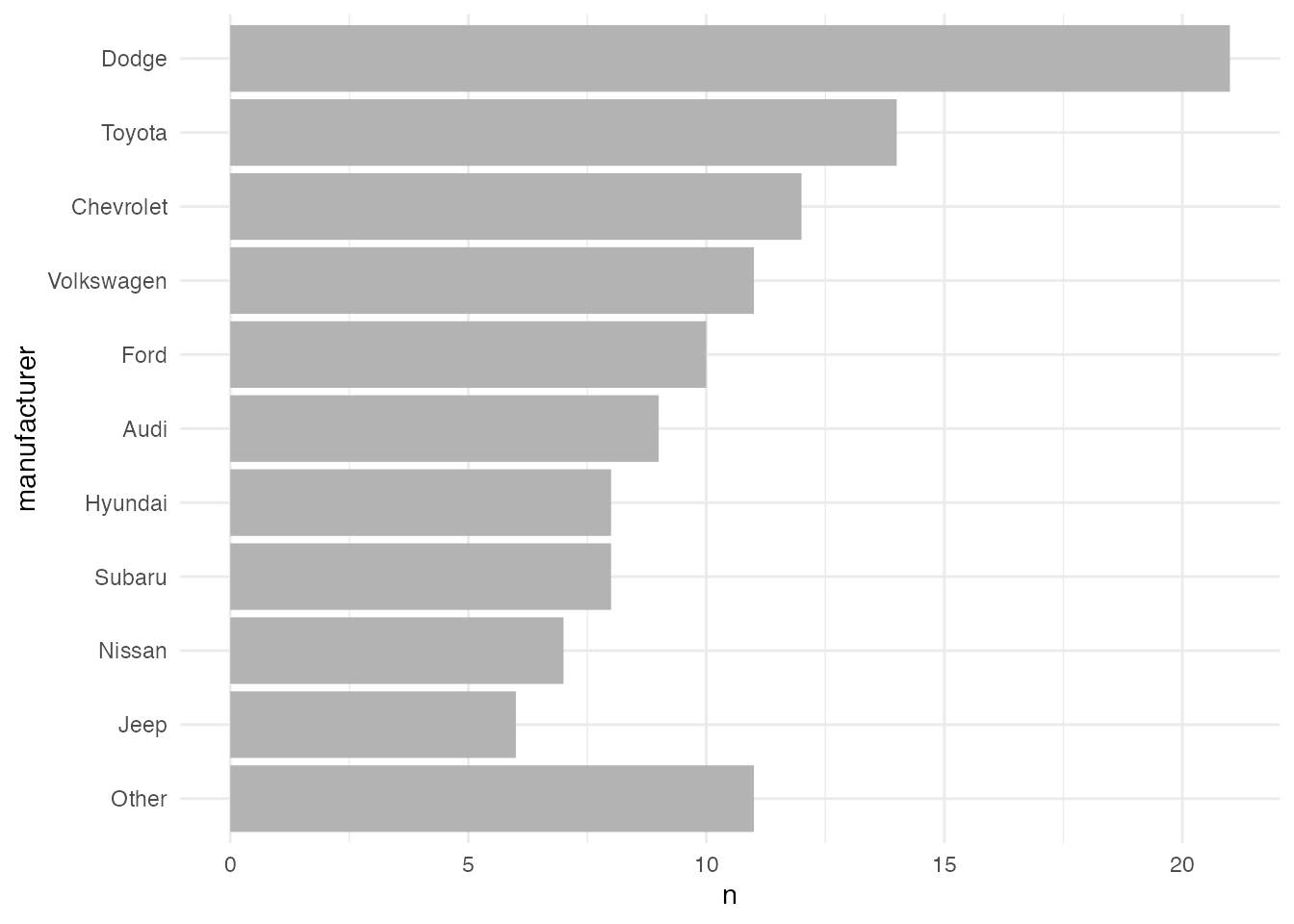##### How to Calculate the Percentage Values

We can go both routes, either creating the labels first or on the fly. However, creating the bars and labels with the help of `geom_bar()` and `stat_summary(geom = "text")` is a bit more difficult and I prefer to build a temporary data frame for that task. The benefit is that you always can control and check the output, i.e. the sorting of the factor and the formatting of the labels.

Here are two ways how to quickly add the percentage labels to your data set. The percentage can be easily calculated by dividing the number of cars per manufacturer `n` by the total number of cars `sum(n)`, times 100. `sprintf()` is a handy function to format text and variables. `sprintf()` allows you to include for example leading spaces (not important here but useful for left-aligned labels) and zero digits (e.g. `12.0%` instead of `12%` which is useful here). The syntax is likely confusing for you because it relies on the C library of the same name. Here, we want to retrieve 4 characters in total, 1 of the to the right of the decimal. See here for more about the parameters one can use. Using `paste0`, or alternatively the `glue()` function from the `{glue}` package, we add the percentage symbol to that number.

``````mpg_sum <- mpg_sum |>
## add percentage label with `sprintf()`
dplyr::mutate(perc = paste0(sprintf("%4.1f", n / sum(n) * 100), "%"))

mpg_sum``````
``````## # A tibble: 11 × 3
##    manufacturer     n perc
##    <fct>        <int> <chr>
##  1 Dodge           21 "17.9%"
##  2 Toyota          14 "12.0%"
##  3 Chevrolet       12 "10.3%"
##  4 Volkswagen      11 " 9.4%"
##  5 Other           11 " 9.4%"
##  6 Ford            10 " 8.5%"
##  7 Audi             9 " 7.7%"
##  8 Hyundai          8 " 6.8%"
##  9 Subaru           8 " 6.8%"
## 10 Nissan           7 " 6.0%"
## 11 Jeep             6 " 5.1%"``````

One could also use the `percent()` function from the `{scales}` package. The accuracy determines the number of digits (here `.1`) and we can similarly add the leading white space by setting `trim` to `FALSE`.

``````mpg_sum |>
## add percentage label with `scales::percent()`
dplyr::mutate(perc = scales::percent(n / sum(n), accuracy = .1, trim = FALSE))``````
``````## # A tibble: 11 × 3
##    manufacturer     n perc
##    <fct>        <int> <chr>
##  1 Dodge           21 "17.9%"
##  2 Toyota          14 "12.0%"
##  3 Chevrolet       12 "10.3%"
##  4 Volkswagen      11 " 9.4%"
##  5 Other           11 " 9.4%"
##  6 Ford            10 " 8.5%"
##  7 Audi             9 " 7.7%"
##  8 Hyundai          8 " 6.8%"
##  9 Subaru           8 " 6.8%"
## 10 Nissan           7 " 6.0%"
## 11 Jeep             6 " 5.1%"``````

So let’s add the prepared percentage label to our bar graph with `geom_text()`:

``````ggplot(mpg_sum, aes(x = n, y = manufacturer)) +
geom_col(fill = "gray70") +
geom_text(aes(label = perc)) +
theme_minimal()``````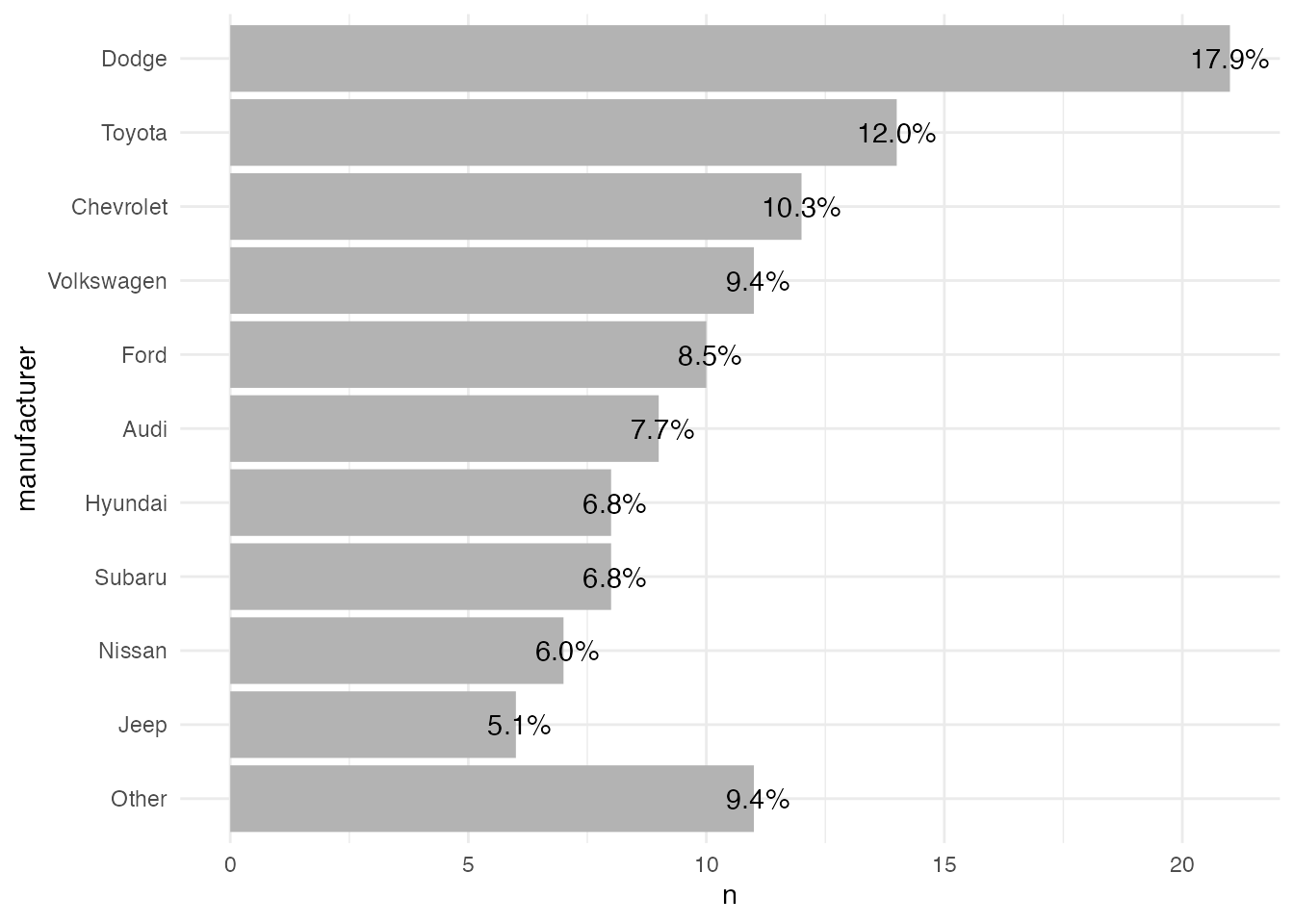And in case you want to add some more description to one of the bars, you can use an `if_else()` (or an `ifelse()`) statement like this:

``````mpg_sum <- mpg_sum |>
dplyr::mutate(
perc = paste0(sprintf("%4.1f", n / sum(n) * 100), "%"),
## customize label for the first category
perc = if_else(row_number() == 1, paste(perc, "of all car models"), perc)
)

ggplot(mpg_sum, aes(x = n, y = manufacturer)) +
geom_col(fill = "gray70") +
geom_text(aes(label = perc)) +
## make sure labels doesn't get cut
scale_x_continuous(limits = c(NA, 24)) +
theme_minimal()``````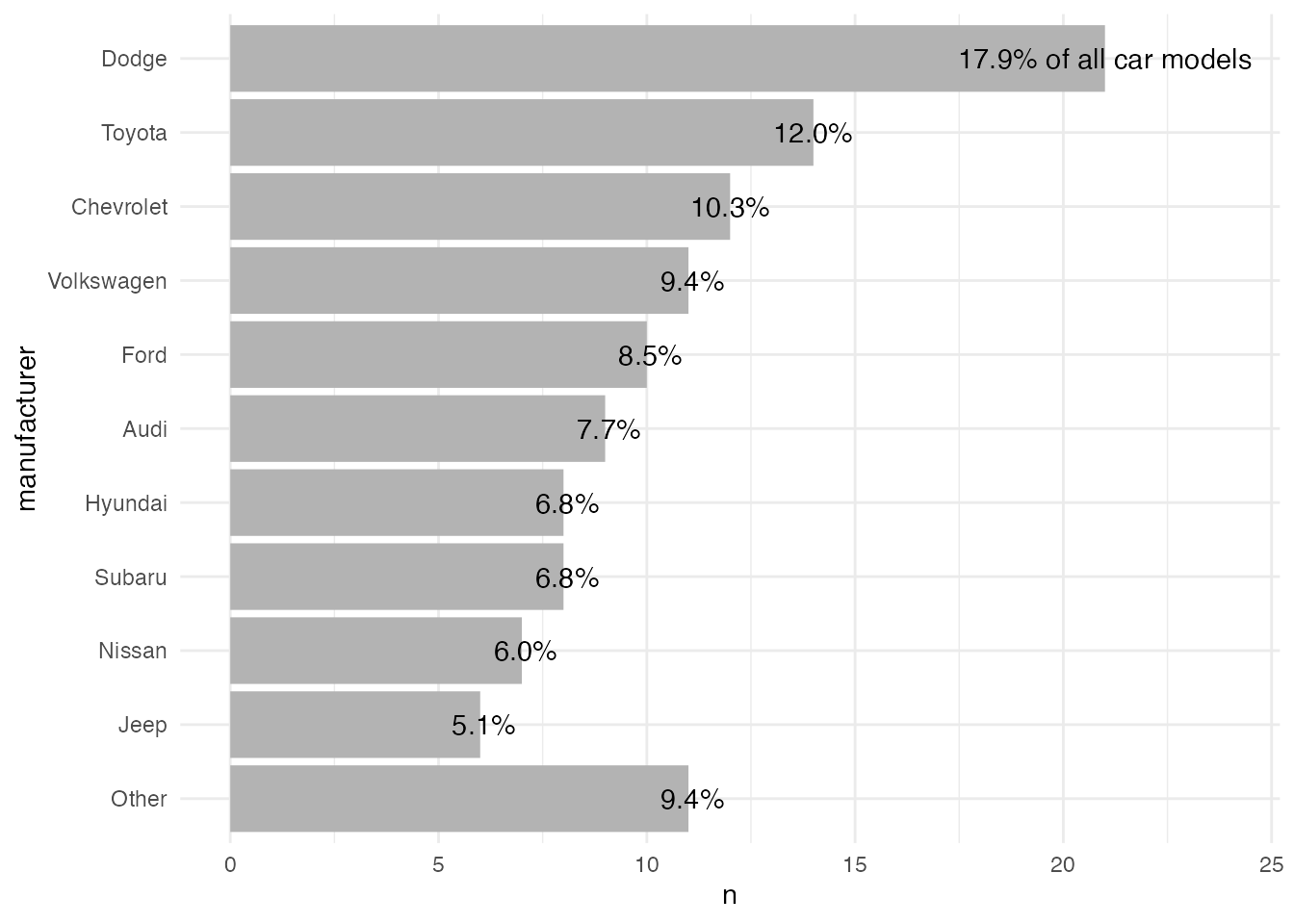To illustrate how to create and place the labels on the fly, here is an example with labels showing counts per manufacturer (with percentage labels it gets a bit more complicated). We use `geom_bar()` instead of `geom_col()` which takes not two but only one variable and calculates counts by default. To add the labels, we again use `geom_text()` but this time we overwrite the default statistical transformation `stat = "identity"` with `stat = "count"` (the same as the default for `geom_bar()`).

``````## prepare non-aggregated data set with lumped and ordered factors
mpg_fct <- mpg |>
dplyr::filter(year == 2008) |>
dplyr::mutate(
## add count to calculate percentages later
total = dplyr::n(),
## turn into lumped factors with capitalized names
manufacturer = stringr::str_to_title(manufacturer),
manufacturer = forcats::fct_lump(manufacturer, n = 10),
## order factor levels by number, put "Other" to end
manufacturer = forcats::fct_rev(forcats::fct_infreq(manufacturer)),
manufacturer = forcats::fct_relevel(manufacturer, "Other", after = 0)
)

ggplot(mpg_fct, aes(x = manufacturer)) +
geom_bar(fill = "gray70") +
geom_text(
stat = "count",
aes(label = ..count..)
) +
## rotate plot
coord_flip()  +
theme_minimal()``````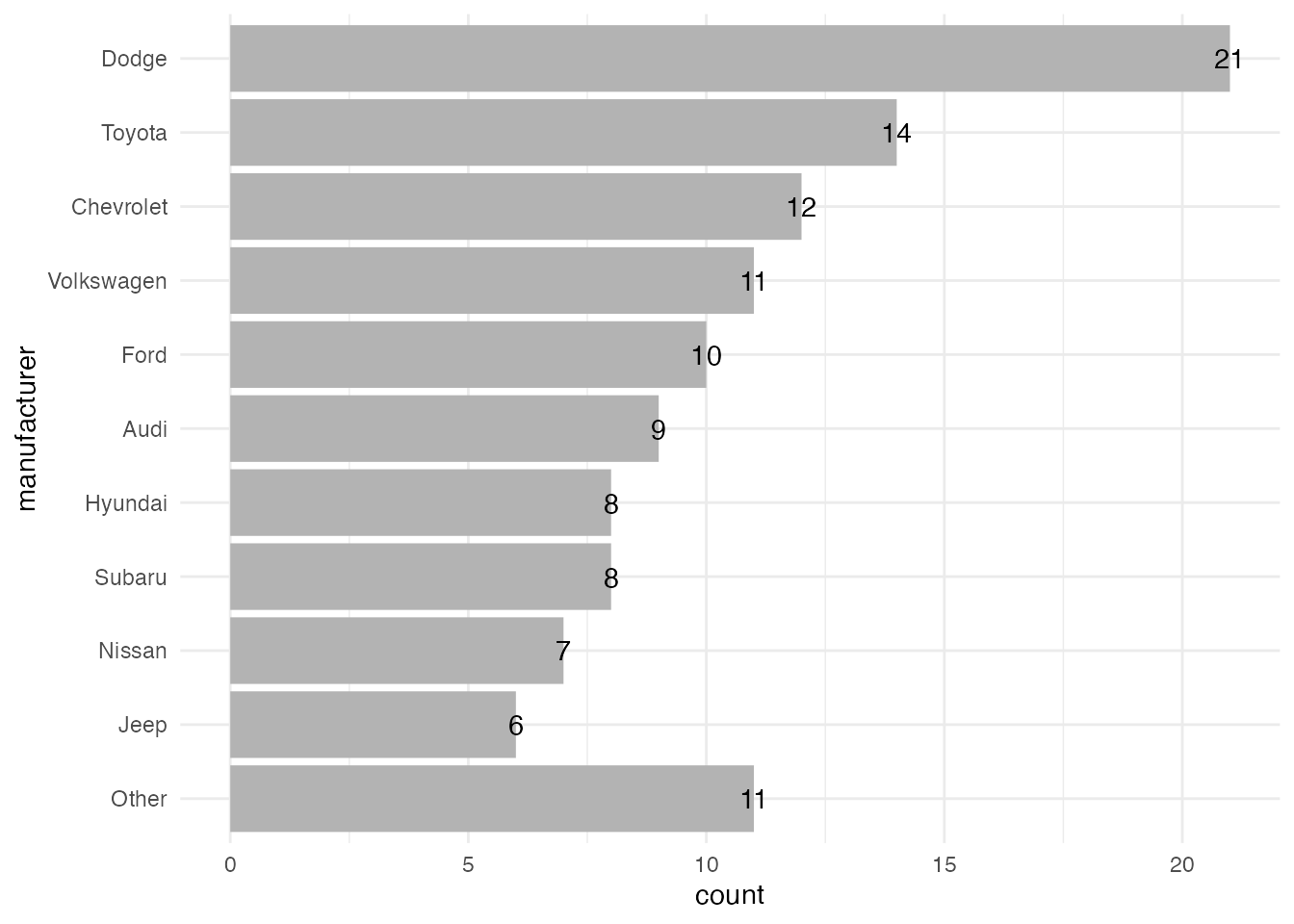As you can see, the default settings of `geom_text()` place the labels exactly on the border. To make it look good, we need to adjust the positioning of the labels. So let’s move on to the next question!

##### How to Position the Percentage Labels Inside the Bars

The `geom_text()` function comes with arguments that help you to align and position text labels:

• `hjust` and `vjust`: the horizontal and vertical justification to align text.
• `nudge_x` and `nudge_y`: the horizontal and vertical adjustment to offset text from points.

To put the labels inside, we first need to right-align the labels with `hjust = 1`. We also add some negative horizontal adjustment via `nudge_x = -.5` to add some spacing between the end of the bar and the label.

``````ggplot(mpg_sum, aes(x = n, y = manufacturer)) +
geom_col(fill = "gray70") +
geom_text(
aes(label = perc),
## make labels left-aligned
hjust = 1, nudge_x = -.5
) +
theme_minimal()``````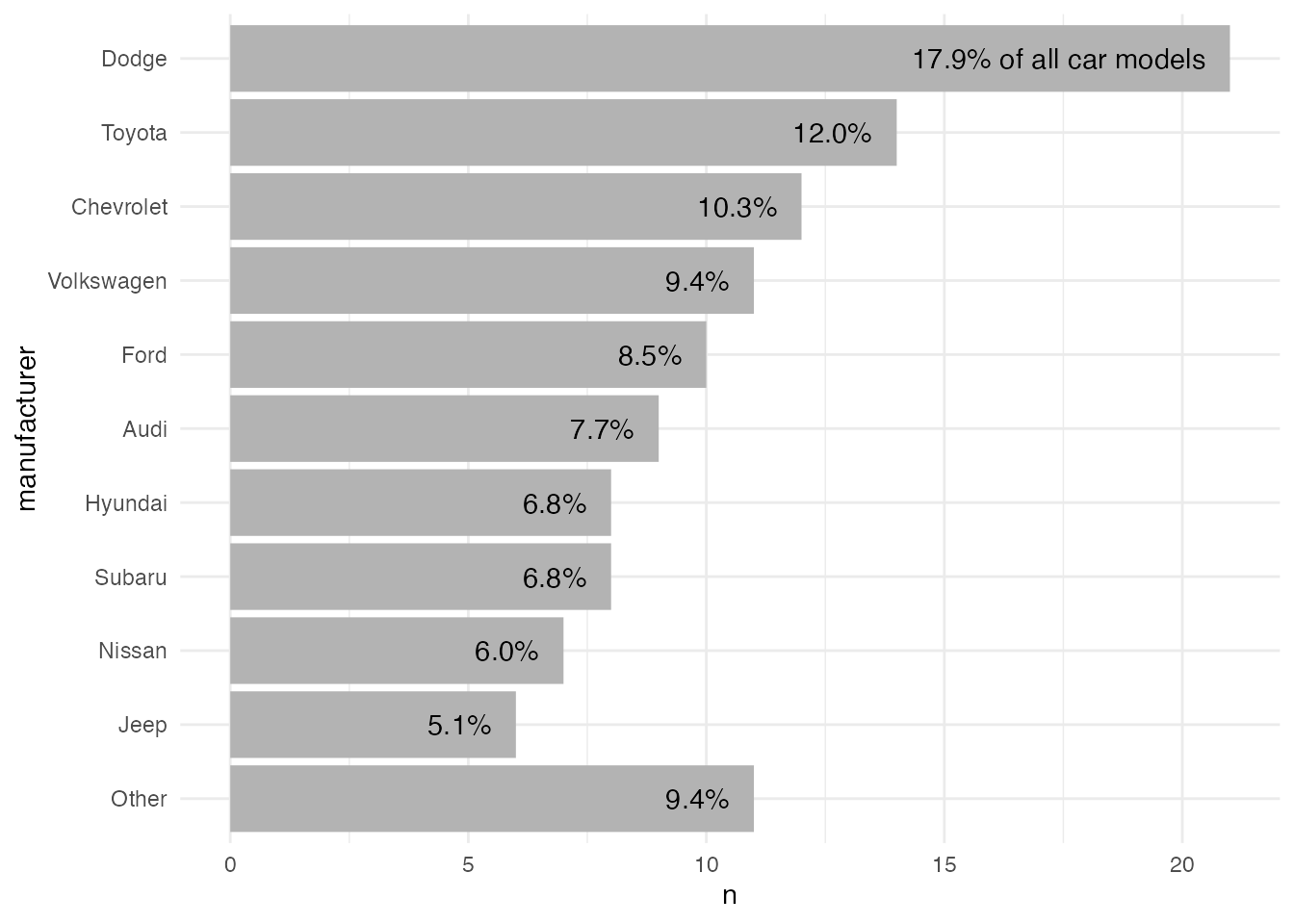In case you want to put the next to the bars, you often need to adjust the plot margin and/or the limits to avoid that the labels are cut off. The drawback of using limits is that you have to define them manually. Thus, I prefer to use the first approach. You can make sure that labels are not truncated by the panel by adding `clip = "off"` to any coordinate system.

💁 Expand to see examples with labels next to the bars.

Increase space on the right via `theme(plot.margin)`:

``````ggplot(mpg_sum, aes(x = n, y = manufacturer)) +
geom_col(fill = "gray70") +
geom_text(
aes(label = perc),
hjust = 0, nudge_x = .5
) +
## make sure labels doesn't get cut, part 1
coord_cartesian(clip = "off") +
theme_minimal() +
## make sure labels doesn't get cut, part 2
theme(plot.margin = margin(r = 120))``````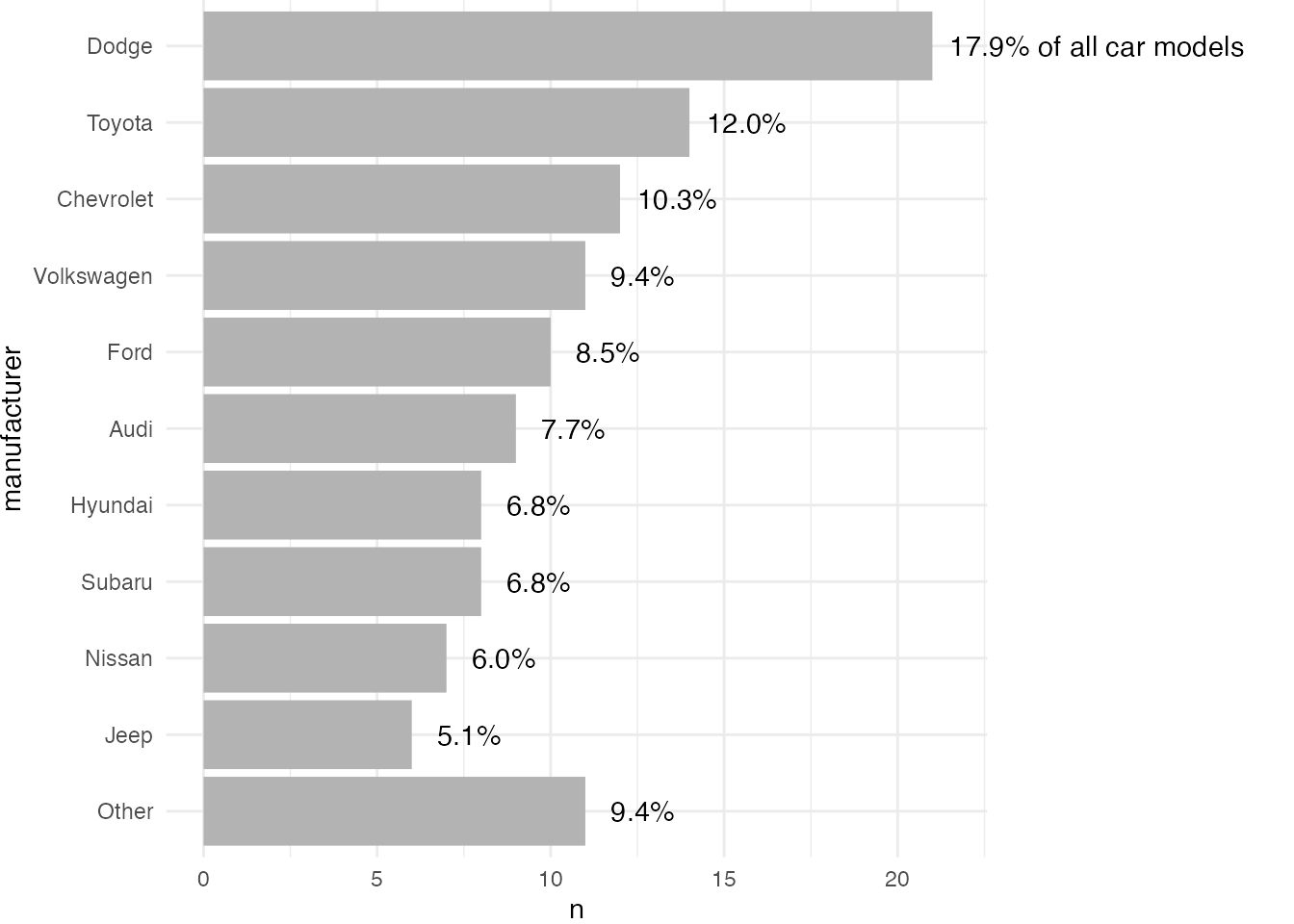Increase space on the right via `scale_x_continuous(limits)`:

``````ggplot(mpg_sum, aes(x = n, y = manufacturer)) +
geom_col(fill = "gray70") +
geom_text(
aes(label = perc),
hjust = 0, nudge_x = .5
) +
## make sure labels doesn't get cut
scale_x_continuous(limits = c(NA, 30)) +
theme_minimal()``````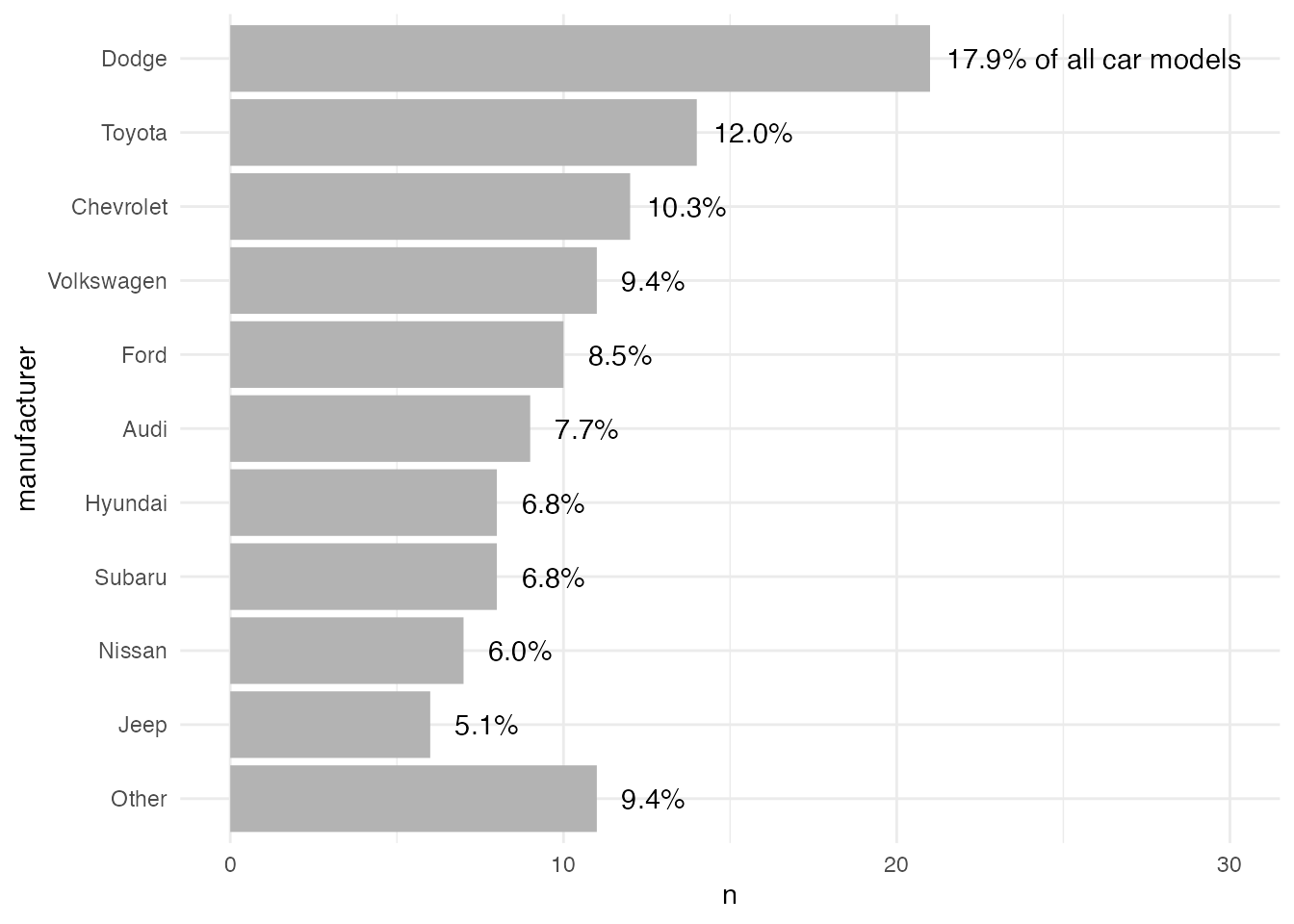##### How to Color the Bars Using Different Colors

Again, there are many ways how to add custom colors. As the first approach, we create the color palette as a vector based on our summarized data set. Let’s pick some colors that are similar to the original plot we started with:

``````## create color palette based on input data
pal <- c(
"gray85",
rep("gray70", length(mpg_sum\$manufacturer) - 4),
"coral2", "mediumpurple1", "goldenrod1"
)``````

In this approach, we create a vector that holds the colors for each of the levels—from the lowest bar to the bar with the most values. We can use the `length` of the manufacturer column for all non-highlighted bars and subtract the number of bars we want to highlight. Here, we have a colorful top 3 and a lighter “Other” category. The vector can then be used in combination with `scale_color|fill_manual()`.

Now, we can use the custom palette to color each bar by mapping `manufacturer` to the bar’s `fill` property and by passing the `pals` vector to `scale_fill_manual()`:

``````ggplot(mpg_sum, aes(x = n, y = manufacturer,
fill = manufacturer)) +
geom_col() +
geom_text(
aes(label = perc),
hjust = 1, nudge_x = -.5
) +
scale_fill_manual(values = pal, guide = "none") +
theme_minimal()``````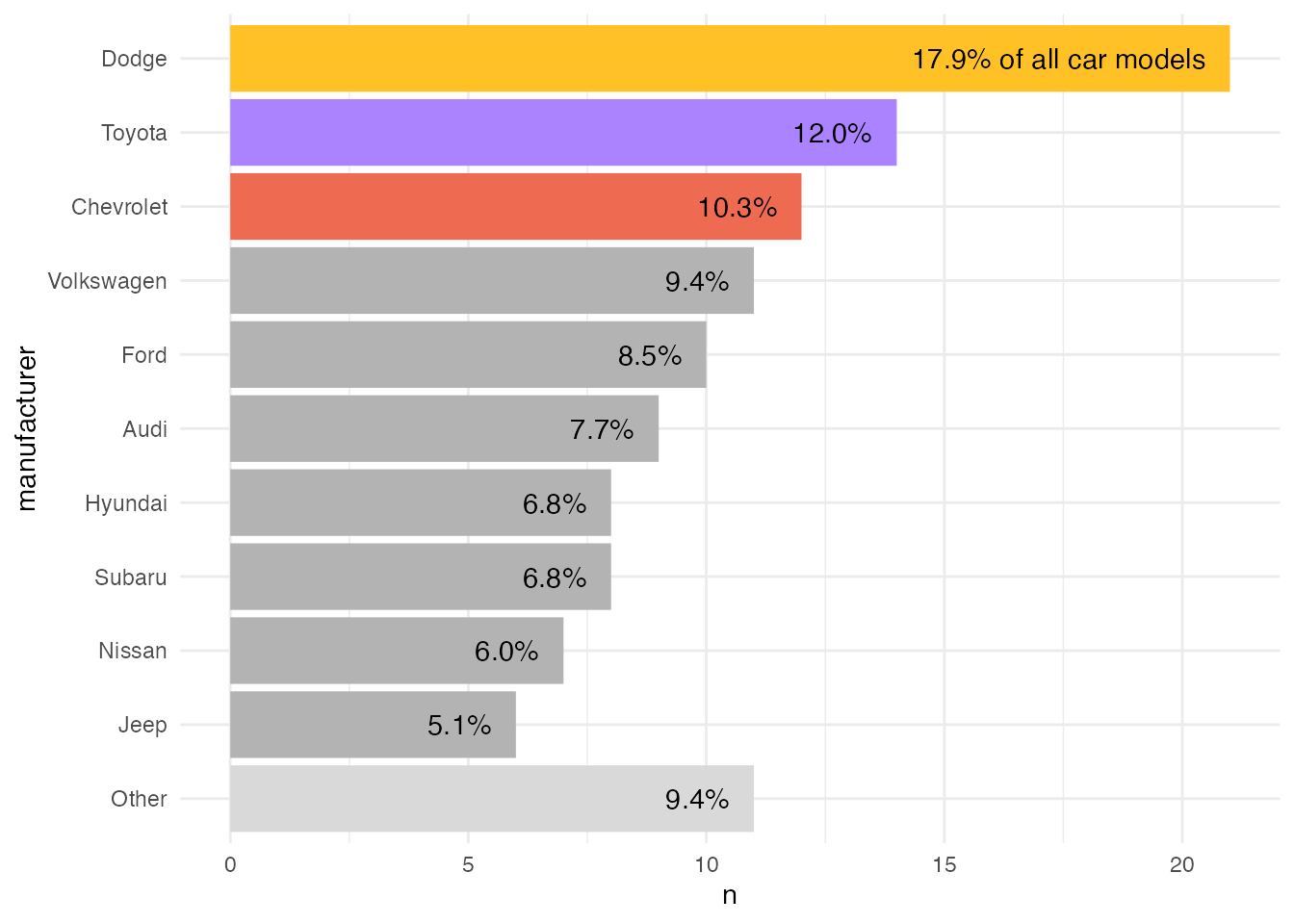One could also add the color to the data set and map the fill to that column and use `scale_fill_identity()`.

``````mpg_sum <-
mpg_sum |>
mutate(
color = case_when(
row_number() == 1 ~ "goldenrod1",
row_number() == 2 ~ "mediumpurple1",
row_number() == 3 ~ "coral2",
manufacturer == "Other" ~ "gray85",
## all others should be gray
TRUE ~ "gray70"
)
)

ggplot(mpg_sum, aes(x = n, y = manufacturer, fill = color)) +
geom_col() +
geom_text(
aes(label = perc),
hjust = 1, nudge_x = -.5
) +
scale_fill_identity(guide = "none") +
theme_minimal()``````This approach is less error-prone since the color is tied to the properties of the data. Thus, in case we update the data, the colors are still applied correctly.

💁 Expand to see that it still works with “updated” data.
``````mpg |>
## use 1999 data now
dplyr::filter(year == 1999) |>
dplyr::mutate(
manufacturer = stringr::str_to_title(manufacturer),
manufacturer = forcats::fct_lump(manufacturer, n = 10)
) |>
dplyr::count(manufacturer, sort = TRUE) |>
dplyr::mutate(
manufacturer = forcats::fct_rev(forcats::fct_inorder(manufacturer)),
manufacturer = forcats::fct_relevel(manufacturer, "Other", after = 0),
perc = paste0(sprintf("%4.1f", n / sum(n) * 100), "%"),
perc = if_else(row_number() == 1, paste(perc, "of all car models"), perc),
color = case_when(
row_number() == 1 ~ "goldenrod1",
row_number() == 2 ~ "mediumpurple1",
row_number() == 3 ~ "coral2",
manufacturer == "Other" ~ "gray85",
TRUE ~ "gray70"
)
) |>
ggplot(aes(x = n, y = manufacturer, fill = color)) +
geom_col() +
geom_text(
aes(label = perc),
hjust = 1, nudge_x = -.5
) +
scale_fill_identity(guide = "none") +
theme_minimal()``````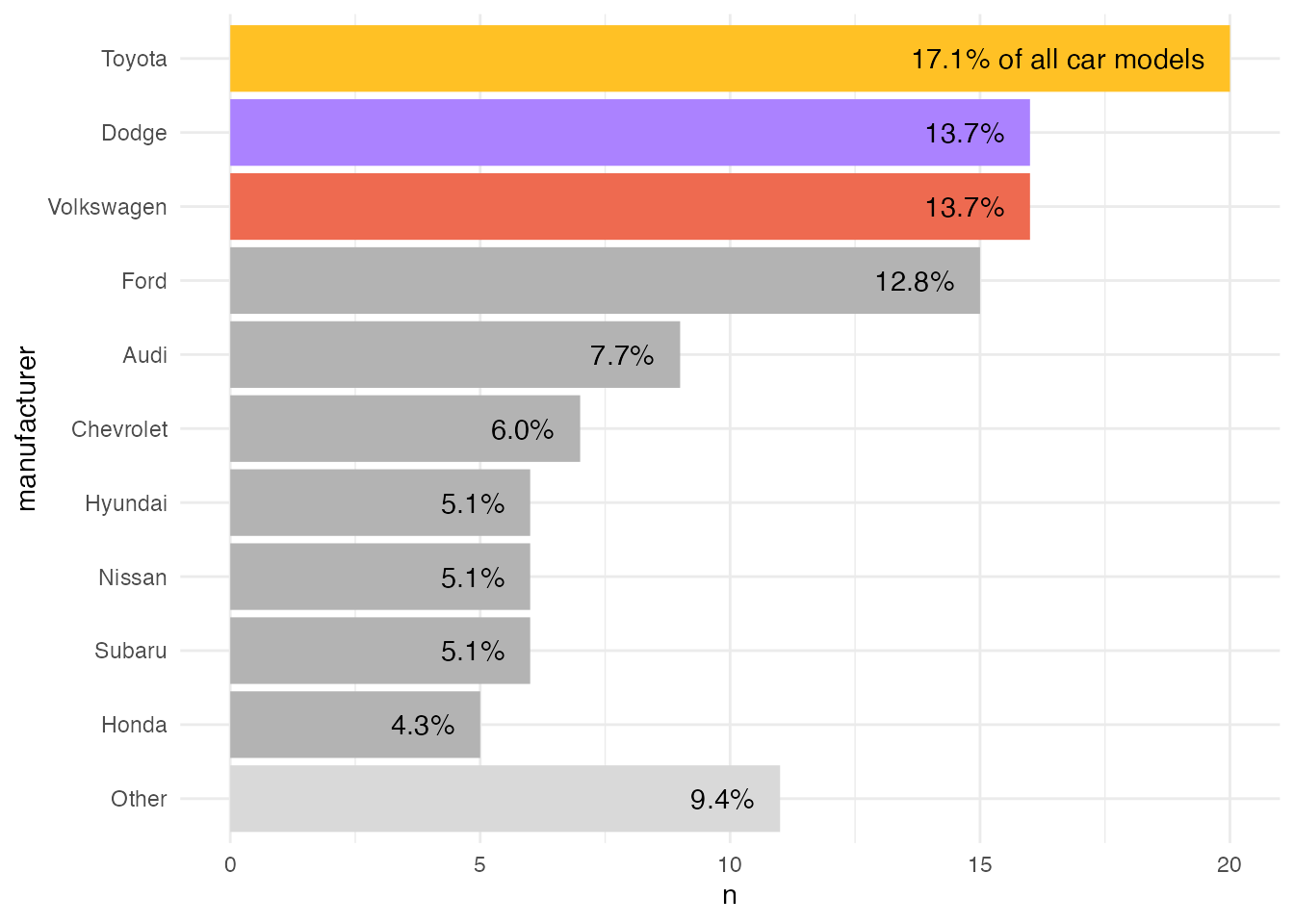Finally, we can adjust the visual appearance a bit, most importantly reduce redundancy. Since I only want to keep the labels on the y axis, I use `theme_void()` and add the axis text afterwards again. Here, I use a custom font for both the axis text and the percentage labels and adjust the font size. (I am not going to cover it here but in case you want to include custom fonts, check the `{systemfonts}` package.)

By default, `{ggplot2}` adds some padding to each axis which results in labels that are a bit off. To decrease the distance between the y axis text and the bars, adjust the expansion argument `expand` in the according scale, here `scale_x_continuous()`. I also add some white space around the plot by setting a `plot.margin` which is of type `element_rect()`.

``````ggplot(mpg_sum, aes(x = n, y = manufacturer, fill = color)) +
geom_col() +
geom_text(
aes(label = perc),
hjust = 1, nudge_x = -.5,
size = 4, fontface = "bold", family = "Fira Sans"
) +
## reduce spacing between labels and bars
scale_x_continuous(expand = c(.01, .01)) +
scale_fill_identity(guide = "none") +
## get rid of all elements except y axis labels + adjust plot margin
theme_void() +
theme(axis.text.y = element_text(size = 14, hjust = 1, family = "Fira Sans"),
plot.margin = margin(rep(15, 4)))``````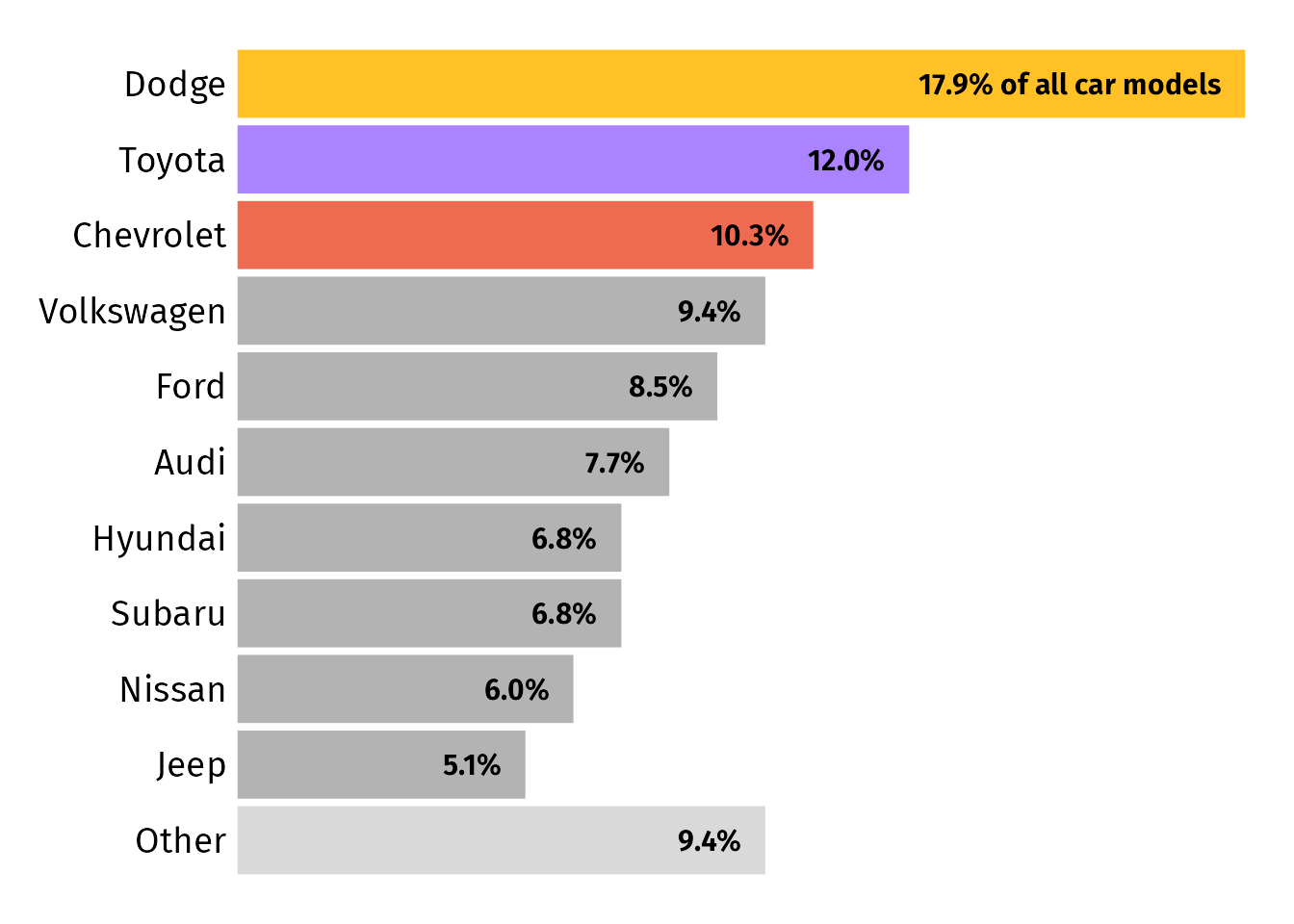You can find the full code to create the final plot in this gist.

##### Alternatives Improving the Accessibility

Update: Some feedback suggested that placing labels inside the bars can hinder accessibility due to contrast issues. I fully agree, so I want to present some approaches to decrease that barrier without the need to increase the white space towards the right when placing labels next to the bars.

Version with label boxes instead of pure text:
We can replace `geom_text()` with `geom_label()` which adds a box around each label. While it doesn’t look as good, the high contrast of black labels on white ground maximizes readability.

``````ggplot(mpg_sum, aes(x = n, y = manufacturer, fill = color)) +
geom_col() +
geom_label(
aes(label = perc),
hjust = 1, nudge_x = -.5,
size = 4, fontface = "bold", family = "Fira Sans",
## turn into white box without outline
fill = "white", label.size = 0
) +
scale_x_continuous(expand = c(.01, .01)) +
scale_fill_identity(guide = "none") +
theme_void() +
theme(
axis.text.y = element_text(size = 14, hjust = 1, family = "Fira Sans"),
plot.margin = margin(rep(15, 4))
)``````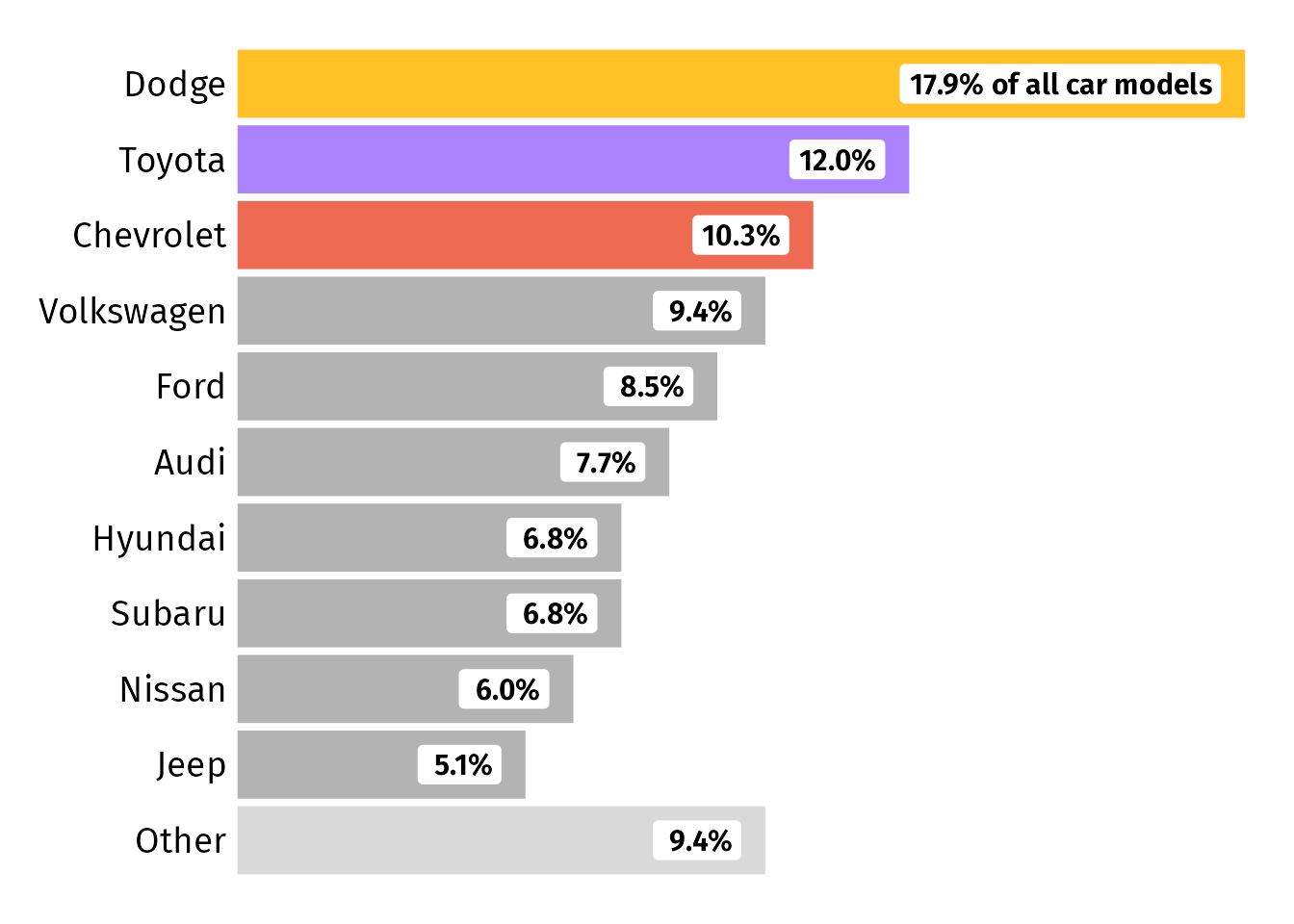Version with different label placement: I like the idea of placing only those labels inside that mess up the aspect ratio due to their length. In our case, that’s only the first entry. We can place the labels differently by mapping a new created column `place` to the `hjust` argument. Since we cannot map a variable to `nudge_x`, we cannot use it to offset the labels. To add some spacing, I simply add some white space to the begin and end of each percentage label.

``````mpg_sum |>
mutate(
## set justification based on data
## so that only the first label is placed inside
place = if_else(row_number() == 1, 1, 0),
## add some spacing to labels since we cant use nudge_x anymore
perc = paste(" ", perc, " ")
) |>
ggplot(aes(x = n, y = manufacturer, fill = color)) +
geom_col() +
geom_text(
aes(label = perc, hjust = place),
size = 4, fontface = "bold", family = "Fira Sans"
) +
scale_x_continuous(expand = c(.01, .01)) +
scale_fill_identity(guide = "none") +
theme_void() +
theme(
axis.text.y = element_text(size = 14, hjust = 1, family = "Fira Sans"),
plot.margin = margin(rep(15, 4))
)``````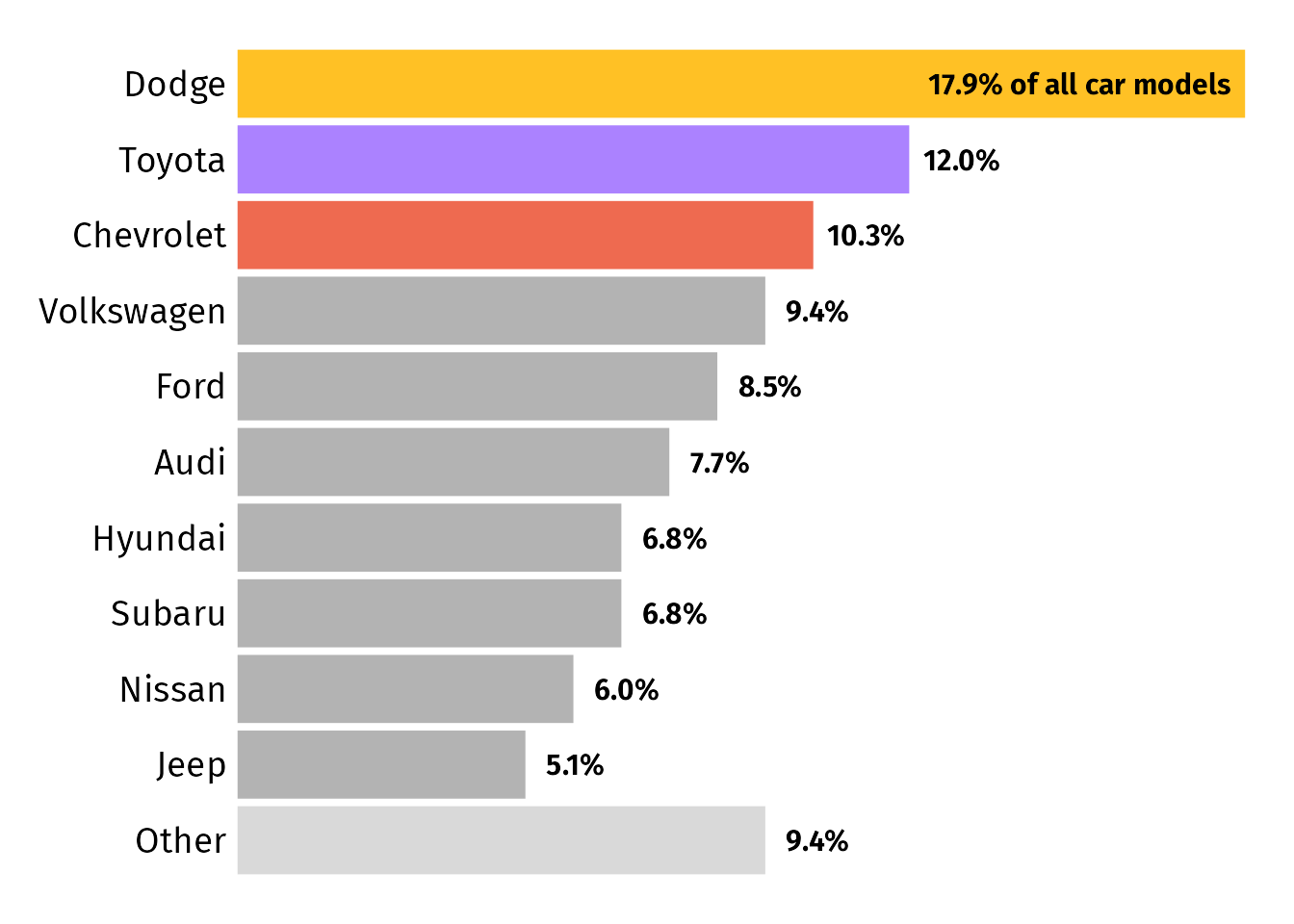Version placing the long label not at the top: We could also add the “of all car models” bit to a bar that is short enough to ensure that the label does not extend beyond the usual width of the plot. In our example, the second bar in our case fulfills this condition:

``````mpg_sum |>
## overwrite old percentage labels
mutate(
perc = paste0(sprintf("%4.1f", n / sum(n) * 100), "%"),
perc = if_else(row_number() == 2, paste(perc, "of all car models"), perc)
) |>
ggplot(aes(x = n, y = manufacturer, fill = color)) +
geom_col() +
geom_text(
aes(label = perc,),
hjust = 0, nudge_x = .5,
size = 4, fontface = "bold", family = "Fira Sans"
) +
## make sure labels doesn't get cut, part 1
coord_cartesian(clip = "off") +
scale_x_continuous(expand = c(.01, .01)) +
scale_fill_identity(guide = "none") +
theme_void() +
theme(
axis.text.y = element_text(size = 14, hjust = 1, family = "Fira Sans"),
## make sure labels doesn't get cut, part 2
plot.margin = margin(15, 30, 15, 15)
)``````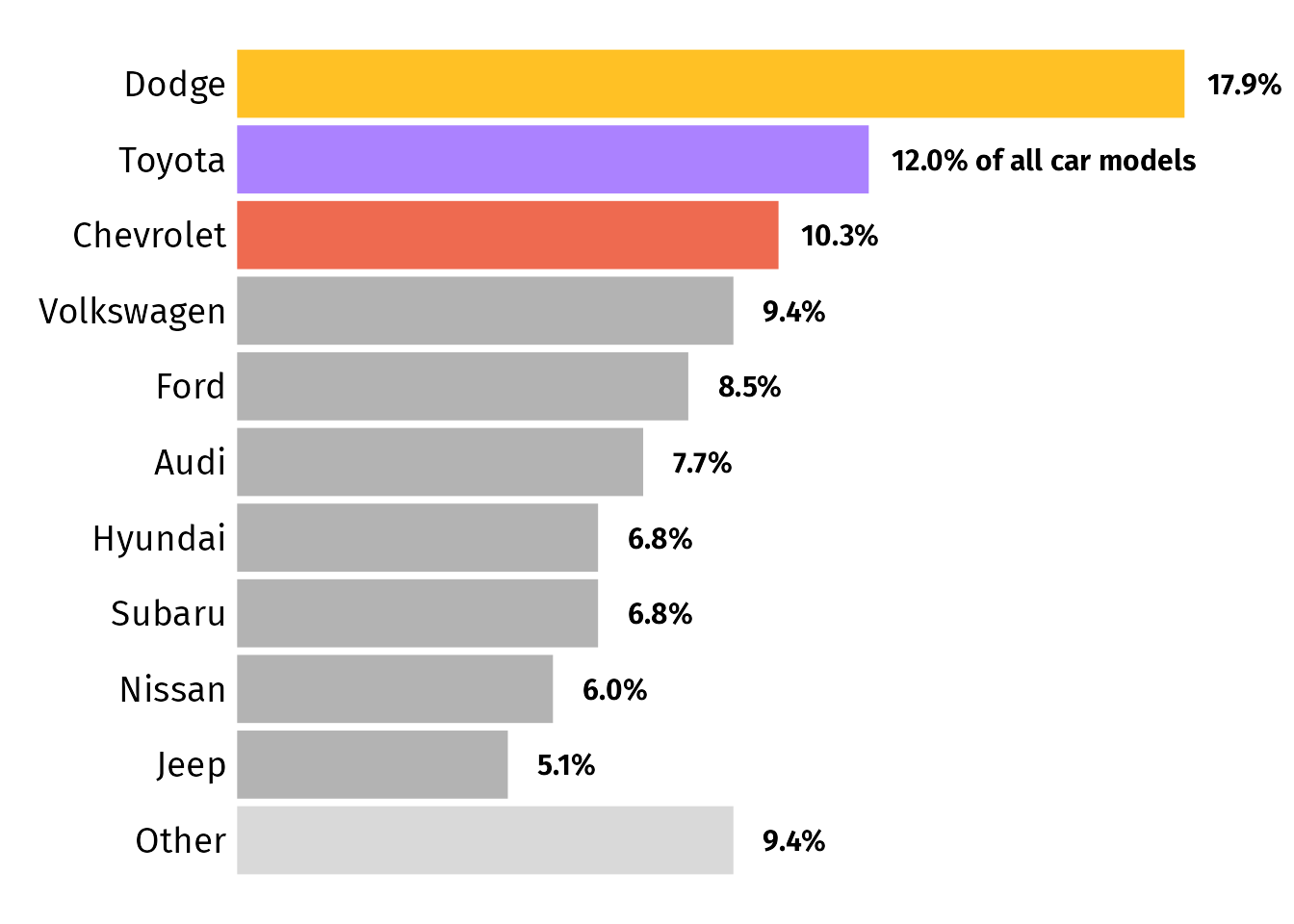Of course, you could add that information also to the title, figure caption or simply leave it out. But that’s not what the request was about 🤷

R Session Info
``````## R version 4.2.3 (2023-03-15)
## Platform: x86_64-apple-darwin17.0 (64-bit)
## Running under: macOS Big Sur ... 10.16
##
## Matrix products: default
## BLAS:   /Library/Frameworks/R.framework/Versions/4.2/Resources/lib/libRblas.0.dylib
## LAPACK: /Library/Frameworks/R.framework/Versions/4.2/Resources/lib/libRlapack.dylib
##
## locale:
##  en_US.UTF-8/en_US.UTF-8/en_US.UTF-8/C/en_US.UTF-8/en_US.UTF-8
##
## attached base packages:
##  stats     graphics  grDevices utils     datasets  methods   base
##
## other attached packages:
##   lubridate_1.9.2   forcats_1.0.0     stringr_1.5.0     dplyr_1.1.2       purrr_1.0.1       readr_2.1.4       tidyr_1.3.0       tibble_3.2.1
##   ggplot2_3.4.2     tidyverse_2.0.0   systemfonts_1.0.4
##
## loaded via a namespace (and not attached):
##   tidyselect_1.2.0  xfun_0.39         bslib_0.5.0       colorspace_2.1-0  vctrs_0.6.2       generics_0.1.3    htmltools_0.5.4   yaml_2.3.7
##   utf8_1.2.3        rlang_1.1.1       jquerylib_0.1.4   pillar_1.9.0      glue_1.6.2        withr_2.5.0       lifecycle_1.0.3   munsell_0.5.0
##  blogdown_1.18     gtable_0.3.1      ragg_1.2.5        evaluate_0.20     labeling_0.4.2    knitr_1.42        tzdb_0.3.0        fastmap_1.1.1
##  fansi_1.0.4       highr_0.10        scales_1.2.1      cachem_1.0.7      jsonlite_1.8.4    farver_2.1.1      textshaping_0.3.6 hms_1.1.2
##  digest_0.6.31     stringi_1.7.12    bookdown_0.34     grid_4.2.3        cli_3.6.0         tools_4.2.3       magrittr_2.0.3    sass_0.4.5
##  pkgconfig_2.0.3   ellipsis_0.3.2    timechange_0.2.0  rmarkdown_2.20    rstudioapi_0.14   R6_2.5.1          compiler_4.2.3``````